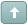Error

 Previous Topic Next Topic
MS Support
#1 Posted : Tuesday, November 5, 2019 6:22:31 PM(UTC)

Groups: Moderators, Registered, Registered Users, Subscribers
Joined: 10/8/2010(UTC)
Posts: 1,631

Thanks: 52 times
Was thanked: 130 time(s) in 126 post(s)

John F. Ehlers' article, “Fourier Series Model of the Market”, explained how to reduce market action to a sinewave using his fourier series analysis. The MetaStock formula for that indicator is below:

# Fourier Series Analysis

Code:
``````x:= Input("fundemental cycle length", 2, 100, 20);
bw:= 0.1; {bandwidth}
L1:= Cos(360/x);
G1:= Cos((bw*360)/x);
S1:= 1/G1 - Sqrt(1/(G1*G1) -1);
L2:= Cos( 360 / (x/2));
G2:= Cos((bw*360)/(x/2));
S2:= 1/G2 - Sqrt(1/(G2*G2) -1);
L3:= Cos( 360 / (x/3));
G3:= Cos((bw*360)/(x/3));
S3:= 1/G3 - Sqrt(1/(G3*G3) -1);
{fundemental band pass}
BP1:= .5*(1-S1)*(C-Ref(C,-2)) + L1*(1+S1)*PREV - S1*Ref(PREV,-1);
Q1:= (x/6.28)*(BP1-Ref(BP1,-1));
{second harmonic band pass}
BP2:= .5*(1-S2)*(C-Ref(C,-2)) + L2*(1+S2)*PREV - S2*Ref(PREV,-1);
Q2:= (x/6.28)*(BP2-Ref(BP2,-1));
{third harmonic band pass}
BP3:= .5*(1-S3)*(C-Ref(C,-2)) + L3*(1+S3)*PREV - S3*Ref(PREV,-1);
Q3:= (x/6.28)*(BP3-Ref(BP3,-1));
{final calculations}
p1:= Sum((BP1*BP1) + (Q1*Q1), x);
p2:= Sum((BP2*BP2) + (Q2*Q2), x);
p3:= Sum((BP3*BP3) + (Q3*Q3), x);
BP1 + Sqrt(p2/p1)*BP2 + Sqrt(p3/p1)*BP3;``````

 Wanna join the discussion?! Login to your Discussions forum accountor Register a new forum account.Users browsing this topic
Forum Jump
You cannot post new topics in this forum.
You cannot reply to topics in this forum.
You cannot delete your posts in this forum.
You cannot edit your posts in this forum.
You cannot create polls in this forum.
You cannot vote in polls in this forum.